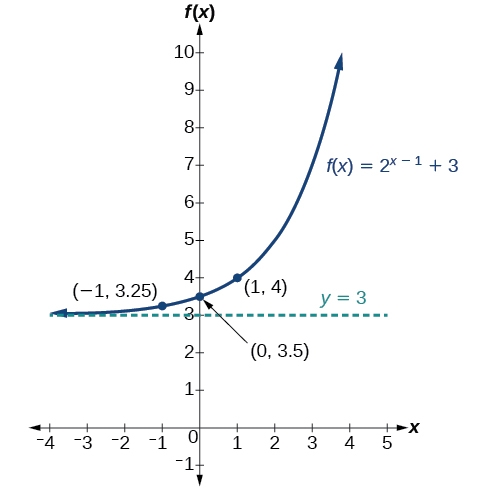6.2 Graphs of exponential functions  (Page 3/6)

 Page 3 / 6

Shifts of the parent function f ( x ) = b x

For any constants $\text{\hspace{0.17em}}c\text{\hspace{0.17em}}$ and $\text{\hspace{0.17em}}d,$ the function $\text{\hspace{0.17em}}f\left(x\right)={b}^{x+c}+d\text{\hspace{0.17em}}$ shifts the parent function $\text{\hspace{0.17em}}f\left(x\right)={b}^{x}$

• vertically $\text{\hspace{0.17em}}d\text{\hspace{0.17em}}$ units, in the same direction of the sign of $\text{\hspace{0.17em}}d.$
• horizontally $\text{\hspace{0.17em}}c\text{\hspace{0.17em}}$ units, in the opposite direction of the sign of $\text{\hspace{0.17em}}c.$
• The y -intercept becomes $\text{\hspace{0.17em}}\left(0,{b}^{c}+d\right).$
• The horizontal asymptote becomes $\text{\hspace{0.17em}}y=d.$
• The range becomes $\text{\hspace{0.17em}}\left(d,\infty \right).$
• The domain, $\text{\hspace{0.17em}}\left(-\infty ,\infty \right),$ remains unchanged.

Given an exponential function with the form $\text{\hspace{0.17em}}f\left(x\right)={b}^{x+c}+d,$ graph the translation.

1. Draw the horizontal asymptote $\text{\hspace{0.17em}}y=d.$
2. Identify the shift as $\text{\hspace{0.17em}}\left(-c,d\right).\text{\hspace{0.17em}}$ Shift the graph of $\text{\hspace{0.17em}}f\left(x\right)={b}^{x}\text{\hspace{0.17em}}$ left $\text{\hspace{0.17em}}c\text{\hspace{0.17em}}$ units if $\text{\hspace{0.17em}}c\text{\hspace{0.17em}}$ is positive, and right $\text{\hspace{0.17em}}c\text{\hspace{0.17em}}$ units if $c\text{\hspace{0.17em}}$ is negative.
3. Shift the graph of $\text{\hspace{0.17em}}f\left(x\right)={b}^{x}\text{\hspace{0.17em}}$ up $\text{\hspace{0.17em}}d\text{\hspace{0.17em}}$ units if $\text{\hspace{0.17em}}d\text{\hspace{0.17em}}$ is positive, and down $\text{\hspace{0.17em}}d\text{\hspace{0.17em}}$ units if $\text{\hspace{0.17em}}d\text{\hspace{0.17em}}$ is negative.
4. State the domain, $\text{\hspace{0.17em}}\left(-\infty ,\infty \right),$ the range, $\text{\hspace{0.17em}}\left(d,\infty \right),$ and the horizontal asymptote $\text{\hspace{0.17em}}y=d.$

Graphing a shift of an exponential function

Graph $\text{\hspace{0.17em}}f\left(x\right)={2}^{x+1}-3.\text{\hspace{0.17em}}$ State the domain, range, and asymptote.

We have an exponential equation of the form $\text{\hspace{0.17em}}f\left(x\right)={b}^{x+c}+d,$ with $\text{\hspace{0.17em}}b=2,$ $\text{\hspace{0.17em}}c=1,$ and $\text{\hspace{0.17em}}d=-3.$

Draw the horizontal asymptote $\text{\hspace{0.17em}}y=d$ , so draw $\text{\hspace{0.17em}}y=-3.$

Identify the shift as $\text{\hspace{0.17em}}\left(-c,d\right),$ so the shift is $\text{\hspace{0.17em}}\left(-1,-3\right).$

Shift the graph of $\text{\hspace{0.17em}}f\left(x\right)={b}^{x}\text{\hspace{0.17em}}$ left 1 units and down 3 units.

The domain is $\text{\hspace{0.17em}}\left(-\infty ,\infty \right);\text{\hspace{0.17em}}$ the range is $\text{\hspace{0.17em}}\left(-3,\infty \right);\text{\hspace{0.17em}}$ the horizontal asymptote is $\text{\hspace{0.17em}}y=-3.$

Graph $\text{\hspace{0.17em}}f\left(x\right)={2}^{x-1}+3.\text{\hspace{0.17em}}$ State domain, range, and asymptote.

The domain is $\text{\hspace{0.17em}}\left(-\infty ,\infty \right);\text{\hspace{0.17em}}$ the range is $\text{\hspace{0.17em}}\left(3,\infty \right);\text{\hspace{0.17em}}$ the horizontal asymptote is $\text{\hspace{0.17em}}y=3.$Given an equation of the form $\text{\hspace{0.17em}}f\left(x\right)={b}^{x+c}+d\text{\hspace{0.17em}}$ for $\text{\hspace{0.17em}}x,$ use a graphing calculator to approximate the solution.

• Press [Y=] . Enter the given exponential equation in the line headed “ Y 1 = ”.
• Enter the given value for $\text{\hspace{0.17em}}f\left(x\right)\text{\hspace{0.17em}}$ in the line headed “ Y 2 = ”.
• Press [WINDOW] . Adjust the y -axis so that it includes the value entered for “ Y 2 = ”.
• Press [GRAPH] to observe the graph of the exponential function along with the line for the specified value of $\text{\hspace{0.17em}}f\left(x\right).$
• To find the value of $\text{\hspace{0.17em}}x,$ we compute the point of intersection. Press [2ND] then [CALC] . Select “intersect” and press [ENTER] three times. The point of intersection gives the value of x for the indicated value of the function.

Approximating the solution of an exponential equation

Solve $\text{\hspace{0.17em}}42=1.2{\left(5\right)}^{x}+2.8\text{\hspace{0.17em}}$ graphically. Round to the nearest thousandth.

Press [Y=] and enter $\text{\hspace{0.17em}}1.2{\left(5\right)}^{x}+2.8\text{\hspace{0.17em}}$ next to Y 1 =. Then enter 42 next to Y2= . For a window, use the values –3 to 3 for $\text{\hspace{0.17em}}x\text{\hspace{0.17em}}$ and –5 to 55 for $\text{\hspace{0.17em}}y.\text{\hspace{0.17em}}$ Press [GRAPH] . The graphs should intersect somewhere near $\text{\hspace{0.17em}}x=2.$

For a better approximation, press [2ND] then [CALC] . Select [5: intersect] and press [ENTER] three times. The x -coordinate of the point of intersection is displayed as 2.1661943. (Your answer may be different if you use a different window or use a different value for Guess? ) To the nearest thousandth, $\text{\hspace{0.17em}}x\approx 2.166.$

Solve $\text{\hspace{0.17em}}4=7.85{\left(1.15\right)}^{x}-2.27\text{\hspace{0.17em}}$ graphically. Round to the nearest thousandth.

$x\approx -1.608$

Graphing a stretch or compression

While horizontal and vertical shifts involve adding constants to the input or to the function itself, a stretch or compression occurs when we multiply the parent function $\text{\hspace{0.17em}}f\left(x\right)={b}^{x}\text{\hspace{0.17em}}$ by a constant $\text{\hspace{0.17em}}|a|>0.\text{\hspace{0.17em}}$ For example, if we begin by graphing the parent function $\text{\hspace{0.17em}}f\left(x\right)={2}^{x},$ we can then graph the stretch, using $\text{\hspace{0.17em}}a=3,$ to get $\text{\hspace{0.17em}}g\left(x\right)=3{\left(2\right)}^{x}\text{\hspace{0.17em}}$ as shown on the left in [link] , and the compression, using $\text{\hspace{0.17em}}a=\frac{1}{3},$ to get $\text{\hspace{0.17em}}h\left(x\right)=\frac{1}{3}{\left(2\right)}^{x}\text{\hspace{0.17em}}$ as shown on the right in [link] .

A laser rangefinder is locked on a comet approaching Earth. The distance g(x), in kilometers, of the comet after x days, for x in the interval 0 to 30 days, is given by g(x)=250,000csc(π30x). Graph g(x) on the interval [0, 35]. Evaluate g(5)  and interpret the information. What is the minimum distance between the comet and Earth? When does this occur? To which constant in the equation does this correspond? Find and discuss the meaning of any vertical asymptotes.
The sequence is {1,-1,1-1.....} has
how can we solve this problem
Sin(A+B) = sinBcosA+cosBsinA
Prove it
Eseka
Eseka
hi
Joel
June needs 45 gallons of punch. 2 different coolers. Bigger cooler is 5 times as large as smaller cooler. How many gallons in each cooler?
7.5 and 37.5
Nando
find the sum of 28th term of the AP 3+10+17+---------
I think you should say "28 terms" instead of "28th term"
Vedant
the 28th term is 175
Nando
192
Kenneth
if sequence sn is a such that sn>0 for all n and lim sn=0than prove that lim (s1 s2............ sn) ke hole power n =n
write down the polynomial function with root 1/3,2,-3 with solution
if A and B are subspaces of V prove that (A+B)/B=A/(A-B)
write down the value of each of the following in surd form a)cos(-65°) b)sin(-180°)c)tan(225°)d)tan(135°)
Prove that (sinA/1-cosA - 1-cosA/sinA) (cosA/1-sinA - 1-sinA/cosA) = 4
what is the answer to dividing negative index
In a triangle ABC prove that. (b+c)cosA+(c+a)cosB+(a+b)cisC=a+b+c.
give me the waec 2019 questionsByByBy Lakeima RobertsBy Anindyo MukhopadhyayBy